Chemguide: Support for CIE A level Chemistry ``` ``` Learning outcome 25: Equilibria 25.1: Acids and bases ``` ``` Learning outcomes 25.1.3 and 25.1.4 These statements include most of the calculations relating to strong and weak acids, and strong bases. CIE don't want calculations involving weak bases. Before you go on, you should find and read the statements in your copy of the syllabus. ``` ``` Explaining the terms pH, Ka, pKa, Kw Start by reading the page about strong and weak acids, revising what you already know, but concentrating on the way that pH, Ka and pKa are defined. Next read the page about the ionic product for water. Then read the first part of the page about strong and weak bases. You need to know what weak bases are, but CIE won't ask you to do any calculations involving them, so you can ignore everything after the red warning box towards the bottom of the page. ``` ``` In their teacher support material, CIE sensibly say that these are all best explained in terms of their mathematical definitions: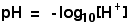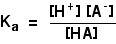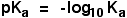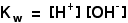If you are asked for a definition or an explanation for one of these in an exam, that should be all you need to write. ``` ``` Calculations involving pH, Ka, pKa, Kw These calculations are covered in detail in my chemistry calculations book, and are also done well in the CIE Chemistry Coursebook. What follows is only a brief introduction, and you will need to use another source for practice questions. ``` ``` Interconverting [H+] and pH, and Ka and pKa All you have to do is use the log button on your calculator and then reverse the sign of the answer to allow for the negative sign in the pH definition. It doesn't matter whether you are talking about a strong or a weak acid, the conversion of hydrogen ion concentration into pH is exactly the same. If you are converting Ka into pKa, you do the same thing. Use the log button on your calculator, and then reverse the sign of the answer. All the pKa values you will meet will be positive. Note:  In my experience, quite a lot of students have never really sorted out how to enter a number like 4 x 10-2 on their calculator. To find out whether you are one of them, enter this number, and then press the equals button. Your answer should, of course, show 0.04. However, I bet that a fair proportion of people reading this will get an answer of 0.4. If that is happening to you, then you are misusing the EXP button. To enter this properly, you enter 4, then press the EXP button, and enter -2 (probably by entering 2 and pressing the +/- button). The EXP button includes the whole of the expression " x 10", and all you have to do is enter the exponent - in this case, -2. If you get this wrong, your answer will probably come out 10 times too big. ``` ``` Students often find reversing this more difficult, usually because they forget about the negative signs in the definitions, and get a silly answer, or because "un-logging" something using a calculator can be a bit confusing. Suppose you were told that the pH of a solution was 4, and asked to find the hydrogen ion concentration. You wouldn't normally write this next bit down, but you have to be able to think it through before you use your calculator:So if your pH is 4, to allow for the negative sign in the definition, you have to enter -4 on your calculator before you can "un-log" it. So you would most probably enter 4 and then press the +/- button. For reasons that you needn't bother about unless you are a mathematician, to un-log a number you use the 10x button. On all the calculators I have ever used, this is always the alternative function on the log key. My current calculator wants me to press shift and then log to un-log a number. If you do this with -4, you should get the answer 1 x 10-4 (or possibly 0.0001). If you forget to put the negative sign in, and un-log 4 rather than -4, you will get a huge answer of 10000. That is 10000 moles per litre. That's just silly, of course - you can't possibly get concentrations that high. ``` ``` pH sums involving strong bases You will find an explanation of how to do that on the page about strong and weak bases. Remember that you only have to be able to calculate the pH of a strong base. ``` ``` pH sums involving weak acids Suppose you have a weak acid HA. A solution of it in water will contain this equilibrium: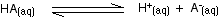The expression for Ka is:If you were given a value of Ka, and were asked to find the hydrogen ion concentration, then this is just an equilibrium calculation. However, we can make it a lot simpler by making two reasonable assumptions. The first assumption, you would probably assume anyway! If the acid is pure, then the concentration of hydrogen ions and the negative ions are the same. In other words:[H+] = [A- ] You might wonder why this is an assumption. What we are doing is ignoring the very, very tiny number of hydrogen ions coming from the ionisation of the water. The second assumption is that, because the acid is weak, the concentration of the acid at equilibrium is the same as the original concentration of the solution. Suppose, for example, that you originally made up the solution to be 1 mol dm-3. We are assuming that so little of the acid is splitting into its ions, that the concentration at equilibrium is still 1 mol dm-3. In truth, of course, it will be less that that, because some of it has split to form ions. But by making this assumption, it makes the maths a lot quicker, and you get an answer which is much the same as if you did it properly. CIE will expect you to make this assumption, which usually gives the same answer for pH to 2 decimal places as if you did it the long way. So, putting this together into a complete calculation: ``` ``` Calculate the pH of a 0.10 mol dm-3 solution of a weak acid, HA, given that Ka = 1.50 x 10-5 mol dm-3. Starting with the expression for Ka:If there is nothing else present in the solution but the acid, the concentrations of hydrogen ions and A- ions are equal, and so this can be simplified to: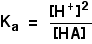Now you can either slot the numbers into this and rearrange it to find a hydrogen ion concentration, or rearrange it first, and then slot the numbers in. Do it whichever way you think best. Normally, I would put the numbers into the equation as it stands, and then rearrange everything. I just find it easier that way. But this time, I am choosing to do it the other way, because of a point I want to make afterwards. Remember that we can assume that the acid concentration is the same as we started with - 0.10 mol dm-3.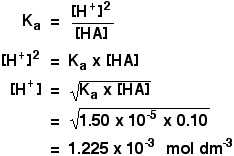Now all you have to do is to convert that into a pH. The number (unrounded) will still be on your calculator, so press the log button, and reverse the sign to allow for the negative sign in the expression for pH. You should get a value for pH of 2.9. Note:  You can't go beyond 2 significant figures because one of the numbers you started with (the concentration) is only quoted to 2 significant figures. ``` ``` Using a formula to do this Many people will try to find the hydrogen ion concentration using a formula, and just slot numbers into it. In the calculation above, we have actually derived the formula which is used. It is the last line before you slot the numbers in. There is another formula which enables you to calculate pH directly using values for pKa and acid concentration. I have no intention of giving you that one! There are two views about this. Some teachers will encourage you to use formulae like these because it is quicker to do in an exam than working it out from scratch. Others (including me) hate using formulae. In my experience, students who use formulae rarely understand what they are doing, and some formulae are quite easy to get confused about. That means that if you mis-remember a formula, you will automatically lose all the marks for that bit of a question. Similarly, if you come up against a case which doesn't quite fit the formula, you are lost. I always work on the assumption that it is better to understand what you are doing even if it takes a little bit longer. Having said that, CIE, in their teacher support material, seem to be encouraging the use of the formula: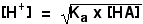Note:  I am really pleased to see that the authors of the CIE Chemistry Coursebook also do these calculations from scratch with no mention of a formula in these examples. Reversing the calculation to find Ka from pH An acid, HA, with a concentration of 0.10 mol dm-3 has a pH of 3.50. Calculate its Ka value. Again starting with the expression for Ka:And, as before, if there is nothing else present in the solution but the acid, the concentrations of hydrogen ions and A- ions are equal, and so this can be simplified to:The first thing to do is to convert the pH into a hydrogen ion concentration. I'm not going to do this for you. Use your calculator! You should get an answer of 3.162 x 10-4. Leave the unrounded answer on your calculator. You have to square this to put it into the Ka expression. Do it! That is now divided by the acid concentration, which for a weak acid you can assume is the same as the stated concentration because so little of it has ionised. If you do that as well, you should get an answer of 1.0 x 10-6. The units are mol dm-3. Note:  You can do this just by starting from the original Ka expression, and doing virtually the whole thing on your calculator as you go along. However, in an exam, write down every step that you are doing. Marks are always available for intermediate steps as well as the final answer. If you make a trivial mistake, you could lose several marks for that one mistake if you didn't show all your working. ``` ``` Go to the Section 25 Menu . . . To return to the list of learning outcomes in Section 25 Go to the CIE Main Menu . . . To return to the list of all the CIE sections Go to Chemguide Main Menu . . . This will take you to the main part of Chemguide. © Jim Clark 2020